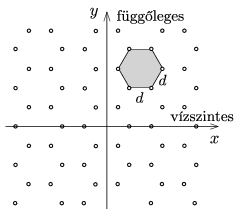Mathematical and Physical Journal
for High Schools
Issued by the MATFUND Foundation
 Already signed up? New to KöMaL?

#Problem P. 4842. (April 2016)

P. 4842. There are small holes on an opaque sheet, the holes positioned in a regular hexagonal grid as shown in the figure. The sheet is illuminated by monochromatic laser light of wavelength $\displaystyle \lambda$ perpendicularly to the sheet.What kind of diffraction pattern can be observed on the screen which is placed at a distance of $\displaystyle L$ from the sheet, if the distance between the holes is $\displaystyle d$? What can be stated about the brightness of the peak intensities with respect to each other? (It can be assumed that $\displaystyle L\ll d \ll \lambda$.)

(6 pont)

Deadline expired on May 10, 2016.

### Statistics:

 6 students sent a solution. 6 points: Ghada Alshalan, Kovács Péter Tamás. 5 points: Büki Máté. 4 points: 1 student. 3 points: 1 student. 0 point: 1 student.

Problems in Physics of KöMaL, April 2016